图像处理 图像相加_opencv处理mat多图像相加 - CSDN
• 在此简单讲述在图像像素相加过程的认识。图形像素相加的是对图像的一种简单的降噪过程。其次，我们知道一幅图像在数学计算过程中，其实就是一个二维矩阵，那么图像的像素相加也就是各个对应点的求和。这就要求我们求...
      在进行图像检测或识别之前，都需要对原始图像进行图像预处理。笔者在此简单描述在图像像素相加过程的认识。图形像素相加是对图像的一种简单降噪过程。其次，我们知道一幅图像在数学计算过程中，其实就是一个二维矩阵，那么图像的像素相加也就是各个对应点的求和。这就要求我们运算的两幅图像的维度必须相同，行和列必须对应相等，否则无法继续，首先我是使用opencv 自带的函数API进行求解，然后通过数学的方式再次进行计算，加深对该运算的理解。

使用API的运行过程：

#include <opencv2/opencv.hpp>
#include <stdio.h>
#include <stdlib.h>

using namespace cv;
using namespace std;

int main(int argc, char** argv)
{

namedWindow("图1", WINDOW_AUTOSIZE);
namedWindow("图2", WINDOW_AUTOSIZE);

imshow("图1",Image_1);
imshow("图2",Image_2);

int Image_1_cols = Image_1.cols;
int Image_1_rows = Image_1.rows;

int Image_2_cols = Image_2.cols;
int Image_2_rows = Image_2.rows;

cout << "Image_1_ cols:" << Image_1_cols <<" "<<"Image_2_ cols:"<< Image_2_cols << endl;
cout << "Image_1_ rows:" << Image_1_rows <<" "<<"Image_2_ rows:"<< Image_2_rows <<endl;

Mat dst;
//dst = Image_1 + Image_2;     //两种方式都可以实现

namedWindow("求和1", WINDOW_AUTOSIZE);
imshow("求和1", dst);
waitKey();
return 1;

}

运行结果如下：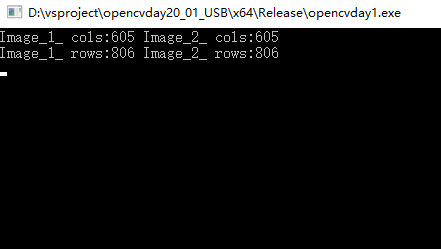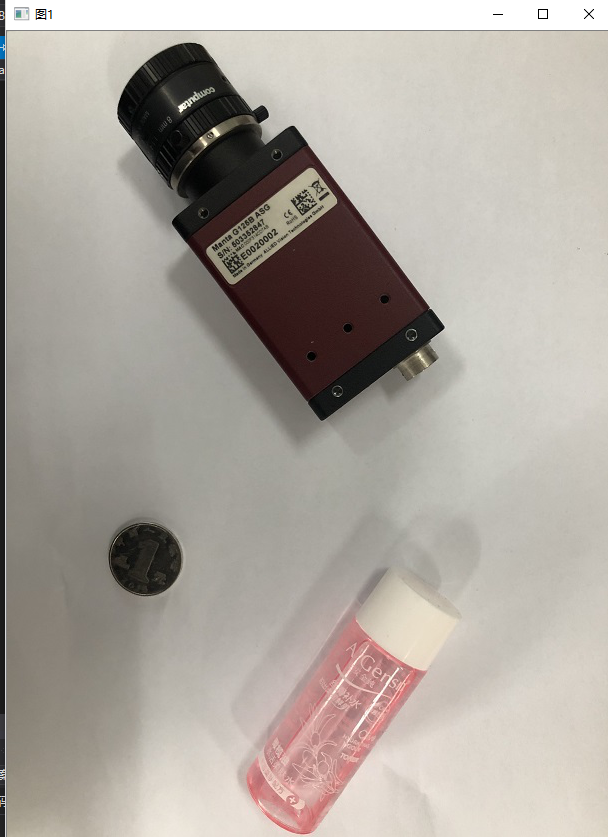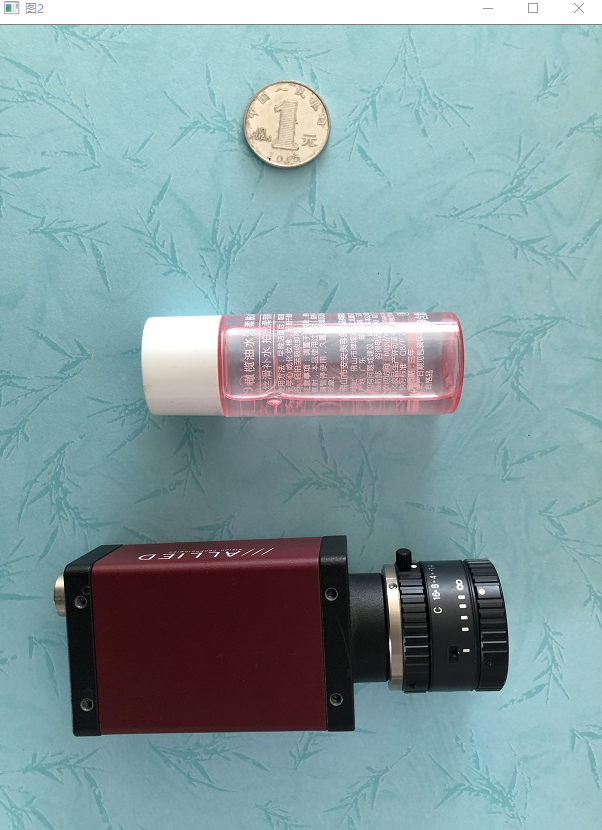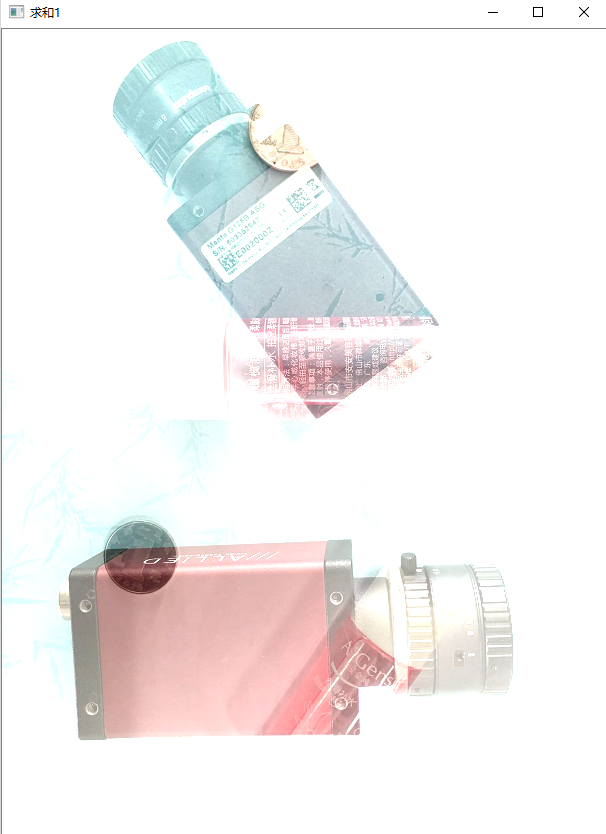使用指针访问像素进行加法运算：

#include <opencv2/opencv.hpp>
#include <stdio.h>
#include <stdlib.h>

using namespace cv;
using namespace std;

int main(int argc, char** argv)
{

namedWindow("图1", WINDOW_AUTOSIZE);
namedWindow("图2", WINDOW_AUTOSIZE);

imshow("图1", Image_1);
imshow("图2", Image_2);

int Image_1_cols = Image_1.cols;
int Image_1_rows = Image_1.rows;

int Image_2_cols = Image_2.cols;
int Image_2_rows = Image_2.rows;

cout << "Image_1_ cols:" << Image_1_cols << " " << "Image_2_ cols:" << Image_2_cols << endl;
cout << "Image_1_ rows:" << Image_1_rows << " " << "Image_2_ rows:" << Image_2_rows << endl;

Mat dst;
dst.create(Image_1.size(), Image_1.type());
//dst = Image_1 + Image_2;     //两种方式都可以实现
int B1, B2, G1, G2, R1, R2;
for (int row = 0; row < Image_1_rows; row++)
{
for (int col = 0; col < Image_1_cols; col++)
{

int B1 = Image_1.at<Vec3b>(row, col);
int G1 = Image_1.at<Vec3b>(row, col);
int R1 = Image_1.at<Vec3b>(row, col);

int B2 = Image_2.at<Vec3b>(row, col);
int G2 = Image_2.at<Vec3b>(row, col);
int R2 = Image_2.at<Vec3b>(row, col);

dst.at<Vec3b>(row, col) = saturate_cast<uchar>(B1 + B2);
dst.at<Vec3b>(row, col) = saturate_cast<uchar>(G1 + G2);
dst.at<Vec3b>(row, col) = saturate_cast<uchar>(R1 + R2);

}
}
namedWindow("求和2", WINDOW_AUTOSIZE);
imshow("求和2", dst);
waitKey(0);
return 1;

}

运行结果如下：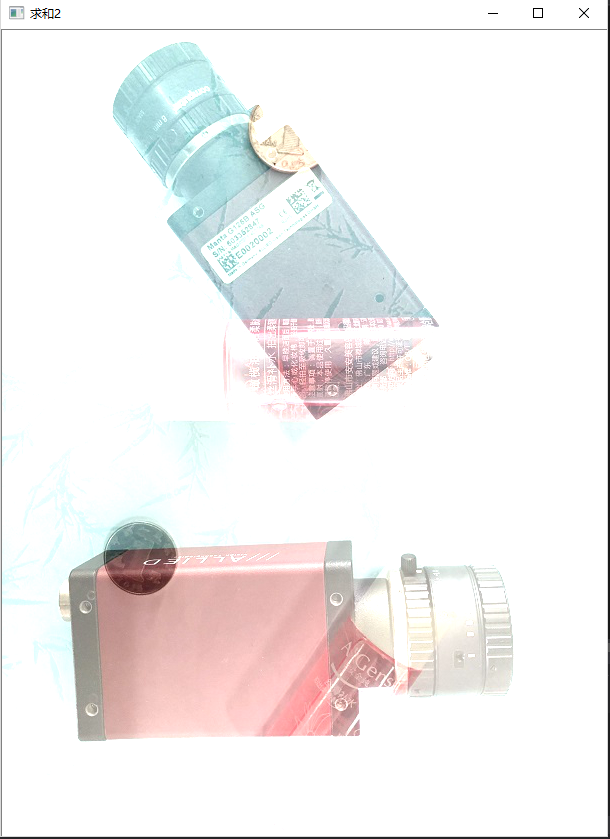展开全文• 看完了数字图像处理后，从头开始使用opencv进行相关内容的实现，使用的环境是VS2013+OpenCV2.4.9 1.图像的加运算 加运算就是两幅图像对应像素的灰度值或彩色分量进行相加。主要有两种用途，一种是消除图像的随机噪声...


看完了数字图像处理后，从头开始使用opencv进行相关内容的实现，使用的环境是VS2013+OpenCV2.4.9
1.图像的加运算
加运算就是两幅图像对应像素的灰度值或彩色分量进行相加。主要有两种用途，一种是消除图像的随机噪声，主要做是讲同一场景的图像进行相加后再取平均；另一种是用来做特效，把多幅图像叠加在一起，再进一步进行处理。
对于灰度图像，因为只有单通道，所以直接进行相应位置的像素加法即可，对于彩色图像，则应该将对应的颜色的分量分别进行相加。
通常来将，两幅或多幅相加的图像的大小和尺寸应该相同。

经过师兄提醒，在这里补充一下，在OpenCV中除了上述使用函数的方法外，还可以直接运用运算符进行操作，例如在求两个图像的和的时候，可以直接写为

result1 = (img1 + img2) / 2;在进行二值化时，再将图像灰度化后，可以直接使用类似语句

result = (img1 > 30);

在实际的使用中，灵活的运用运算符进行图像的操作可以带来很大的方便。

PS：再次感谢师兄的提醒 :D

//本程序实现将两张尺寸相同的图像进行相加//在对像素进行操作时，使用的时迭代器的方法//2017-02-18 #include <iostream>#include <opencv2\core\core.hpp>#include <opencv2\highgui\highgui.hpp> using namespace cv;using namespace std;  int main(){   Mat img1, img2, result1;    img1 = imread("1234.jpg"); img2 = imread("2345.jpg"); result1 = img1.clone(); Mat_<Vec3b>::iterator it = result1.begin<Vec3b>();  //result1初始位置的迭代器 Mat_<Vec3b>::iterator itend = result1.end<Vec3b>(); //result1终止位置的迭代器 Mat_<Vec3b>::iterator it1 = img1.begin<Vec3b>();   //img1初始迭代器    Mat_<Vec3b>::iterator it2 = img2.begin<Vec3b>();    //img2初始迭代器   //进行遍历    for (; it != itend; it++) {       (*it) = ((*it1) + (*it2)) /2;      (*it) = ((*it1) + (*it2)) /2;      (*it) = ((*it1) + (*it2)) /2;      it1++;      it2++;  }   namedWindow("原图1",1); imshow("原图1", img1);   namedWindow("原图2", 0);    imshow("原图2", img2);   namedWindow("相加后的图像",0);  imshow("相加后的图像", result1); Mat result2 = result1.clone();  addWeighted(img1, 1, img2, 1, 0,result2);    namedWindow("addWeighted");    imshow("addWeighted", result2);    waitKey();  return 0;}

运行结果为：2.图像相减

减法运算就是两幅图像见对象像素的灰度值或彩色分量进行相减，它可以用于目标检测，程序实现还是使用两种方法。

以下为程序部分

//本程序实现两个尺寸相同的图像进行相减的操作//分别使用遍历像素的方法和addWeighted的方法 #include <iostream>#include <opencv2\core\core.hpp>#include <opencv2\highgui\highgui.hpp> using namespace std;using namespace cv;uchar toZero(uchar a);//置零函数，小于零则为0int main(){ Mat imag1, imag2, result1, result2; imag1 = imread("2345.jpg");    imag2 = imread("1234.jpg");    result1 = imag1.clone();    result2 = imag2.clone();        int rowNumber = result1.rows; int colNumber = result1.cols; for (int i = 0; i < rowNumber; i++) {       for (int j = 0; j < colNumber; j++)     {           result1.at<Vec3b>(i, j) = toZero(imag1.at<Vec3b>(i, j) - imag2.at<Vec3b>(i, j));          result1.at<Vec3b>(i, j) = toZero(imag1.at<Vec3b>(i, j) - imag2.at<Vec3b>(i, j));          result1.at<Vec3b>(i, j) = toZero(imag1.at<Vec3b>(i, j) - imag2.at<Vec3b>(i, j));      }   }   //addWeighted方法进行图像相减 addWeighted(imag1, 1, imag2, -1, 0,result2); imshow("原图1", imag1);  imshow("原图2", imag2);  imshow("result1", result1);    imshow("addWeighted", result2);    waitKey();  return 0;} uchar toZero(uchar a){    if (a < 0)        return 0;    else      return a;}
运行结果为：result1貌似有问题，需要进一步进行检查。

3.图像相乘
图像的乘法运算就是将两幅图像对应的灰度值或彩色分量进行相乘。
乘运算的主要作用是抑制图像的某些区域，掩膜值置为1，否则置为0。乘运算有时也被用来实现卷积或相关的运算。
以下为相关程序代码。

//图像的乘运算。//将两幅图像对应的灰度值或彩色分量进行相乘。 #include <iostream>#include <opencv2\core\core.hpp>#include <opencv2\highgui\highgui.hpp> using namespace std;using namespace cv; int main(){    Mat imag1, imag2, result;   imag1 = imread("1234.jpg");    imag2 = imread("2345.jpg");    result = imag1.clone(); int rowNumber = result.rows;  int colNumber = result.cols;  for (int i = 0; i < rowNumber; i++) {       for (int j = 0; j < colNumber; j++)     {           result.at<Vec3b>(i, j) = (imag1.at<Vec3b>(i, j) * imag2.at<Vec3b>(i, j)) % 256;          result.at<Vec3b>(i, j) = (imag1.at<Vec3b>(i, j) * imag2.at<Vec3b>(i, j)) % 256;          result.at<Vec3b>(i, j) = (imag1.at<Vec3b>(i, j) * imag2.at<Vec3b>(i, j)) % 256;      }   }   imshow("原图1", imag1);  imshow("原图2", imag2);  imshow("相乘后的图像", result);  waitKey();  return 0;}实验的结果如下：4.图像相除
图像除运算就是两幅图像对应像素的灰度值或彩色分量进行相除。
简单的出运算可以用于改变图像的灰度级

以下为代码部分

//图像的除法运算//就是讲两幅图像的对应像素的灰度值或彩色分量进行相除。//简单的除运算可以用来改变图像的灰度级。 #include <iostream>#include <opencv2\core\core.hpp>#include <opencv2\highgui\highgui.hpp> using namespace std;using namespace cv; int main(){    Mat imag1, imag2, result;   imag2 = imread("1234.jpg");    imag1 = imread("2345.jpg");    result = imag1.clone();     int rowNumber = result.rows;  int colNumber = result.cols;  for (int i = 0; i < rowNumber; i++) {       for (int j = 0; j < colNumber; j++)     {           result.at<Vec3b>(i, j) = (imag1.at<Vec3b>(i, j) * 1.0) / imag2.at<Vec3b>(i, j);          result.at<Vec3b>(i, j) = (imag1.at<Vec3b>(i, j) * 1.0) / imag2.at<Vec3b>(i, j);          result.at<Vec3b>(i, j) = (imag1.at<Vec3b>(i, j) * 1.0) / imag2.at<Vec3b>(i, j);      }   }   imshow("原图1", imag1);  imshow("原图2", imag2);  imshow("相除后的图像", result);  waitKey();  return 0;}

转载自：https://blog.csdn.net/qq_34784753/article/details/55667671?locationNum=1&fps=1
展开全文• 目录 1 图像加法运算 1.1 Numpy库加法运算 1.2 OpenCV加法运算- add() 1.3 对比实验 2 图像融合-addWeighted() ...3 图像类型转换- cvtColor...目标图像 = 图像1 + 图像2，运算结果进行取模运算。有以下两种情况：...目录

1 图像加法运算

1.1 Numpy库加法运算

1.3 对比实验

3 图像类型转换- cvtColor()

参考资料

1 图像加法运算

1.1 Numpy库加法运算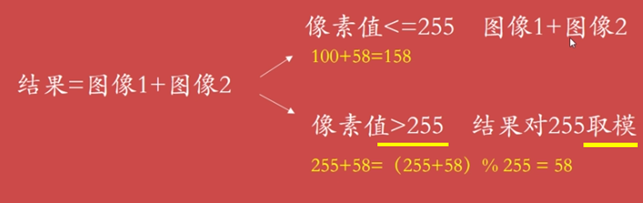Numpy库加法的运算方法为：

目标图像 = 图像1 + 图像2，运算结果进行取模运算。有以下两种情况：

（1）当像素值 <= 255时，结果为“图像1+图像2”，例如：120+48=168

（2）当像素值 > 255时，结果为对255取模的结果，例如：(255+64) % 255=64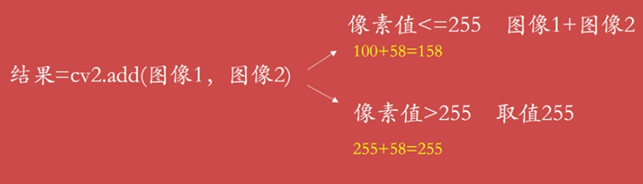OpenCV库实现图像加法运算，方法如下：

目标图像 = cv2.add(图像1, 图像2)  。 此时结果是饱和运算，有以下两种情况：

（1）当像素值 <= 255时，结果为“图像1+图像2”，例如：120+48=168

（2）当像素值 > 255时，结果为255，例如：(255+64) = 255

1.3 对比实验

（1） 两个灰度图像相加

代码如下所示：

# encoding:utf-8
import cv2
import numpy as np
import matplotlib.pyplot as plt

# 读取图片
test = img

# 方法一：Numpy加法运算
result1 = img + test

# 方法二：OpenCV加法运算

# 显示图像
cv2.imshow("original", img)
cv2.imshow("result1_Numpy", result1)
cv2.imshow("result2_OpenCv", result2)

# 等待显示
cv2.waitKey(0)
cv2.destroyAllWindows()

运行结果如下所示：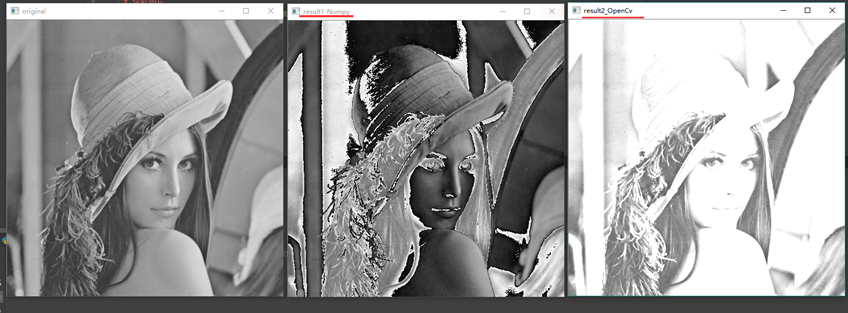注：参与运算的图像大小和类型必须一致。

下面是对彩色图像进行加法运算的结果。

（2）两个彩色图像相加

代码如下：

# encoding:utf-8
import cv2
import numpy as np
import matplotlib.pyplot as plt

# 读取图片
test = img

# 方法一：Numpy加法运算
result1 = img + test

# 方法二：OpenCV加法运算

# 显示图像
cv2.imshow("original", img)
cv2.imshow("result1_Numpy", result1)
cv2.imshow("result2_OpenCv", result2)

运行结果如下：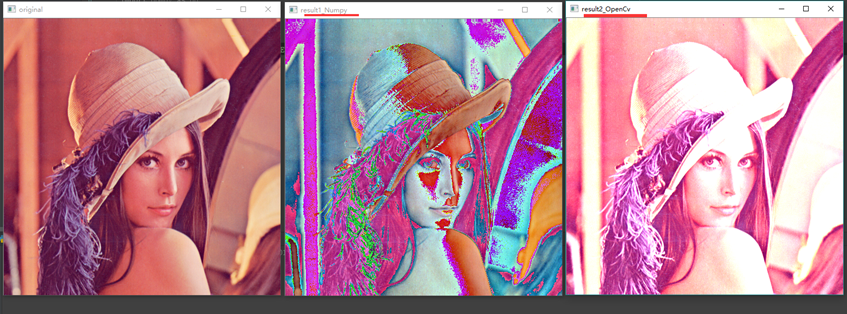图像融合通常是指将2张或2张以上的图像信息融合到1张图像上，融合的图像含有更多的信息，能够更方便人们观察或计算机处理。如下图所示，将两张不清晰的图像融合得到更清晰的图。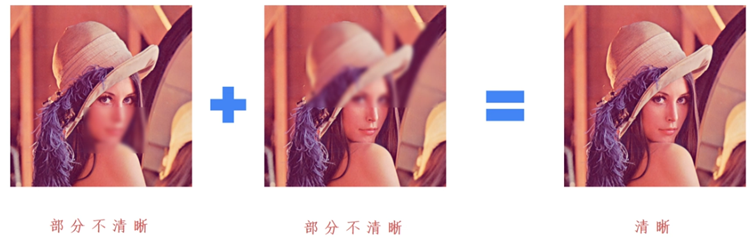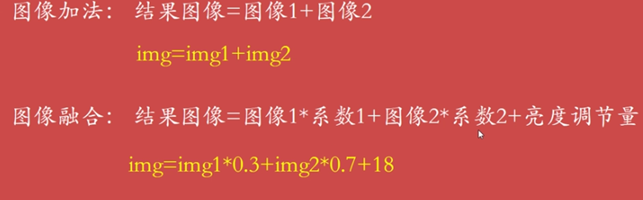如上图所示，图像融合是在图像加法的基础上增加了系数和亮度调节量。

（1）图像加法：目标图像 = 图像1 + 图像2

（2）图像融合：目标图像 = 图像1  系数1 + 图像2  系数2 + 亮度调节量

dst = cv2.addWeighter(scr1, alpha, src2, beta, gamma)

dst = src1  alpha + src2  beta + gamma

其中，参数gamma不能省略。

注：两张融合的图像像素大小需要一致

代码如下所示：

# encoding:utf-8
import cv2
import numpy as np
import matplotlib.pyplot as plt

# 读取图片

# 图像融合
result = cv2.addWeighted(src1, 1, src2, 1, 0)

# 显示图像
cv2.imshow("src1", src1)
cv2.imshow("src2", src2)
cv2.imshow("result", result)

# 等待显示
cv2.waitKey(0)
cv2.destroyAllWindows()


运行结果如下：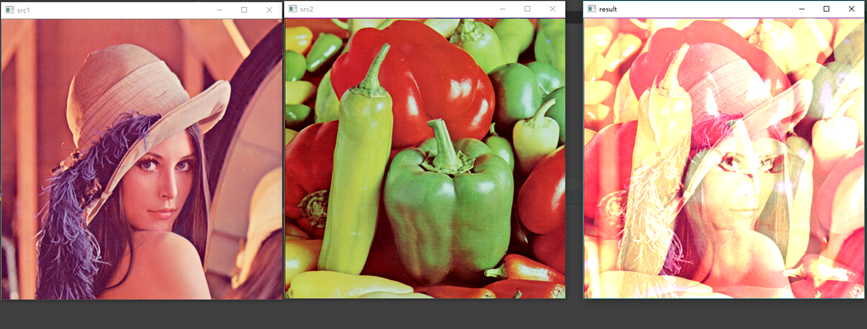（1）设置参数1

result = cv2.addWeighted(src1, 0.8, src2, 0.2, 10)

运行结果如下：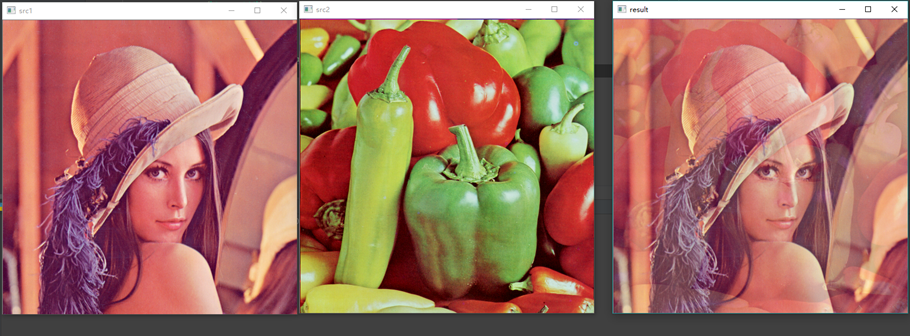（2）设置参数2

result = cv2.addWeighted(src1, 0.2, src2, 0.8, 10)

运行结果如下：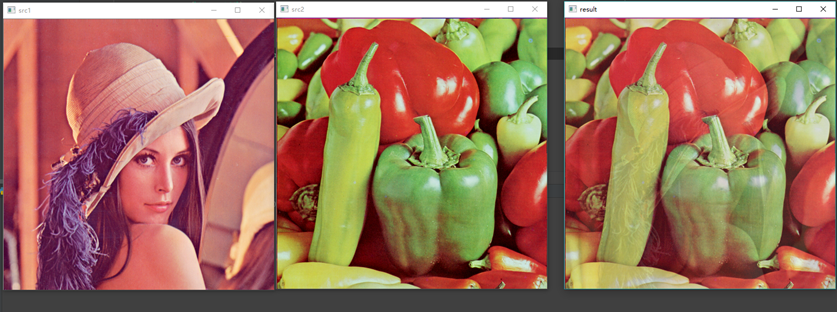3 图像类型转换- cvtColor()

图像类型转换是指将一种类型转换为另一种类型，比如彩色图像转换为灰度图像、BGR图像转换为RGB图像。OPenCV提供了200多种不同类型之间的转换，其中最常用的包括3类，如下：

result = cv2.cvtColor(图像, 参数)

其中，参数有以下三种：

1) cv2.COLOR_BGR2GRAY #彩色转灰度 类似于Matlab 中的 rgb2gray()

2) cv2.COLOR_BGR2RGB

3) cv2.COLOR_GRAY2BGR

（1）彩色图像转灰度图像

代码如下：

# encoding:utf-8
import cv2

# 读取图片

# 图像类型转换
result = cv2.cvtColor(src, cv2.COLOR_BGR2GRAY)

# 显示图像
cv2.imshow("src", src)
cv2.imshow("result", result)

# 等待显示
cv2.waitKey(0)
cv2.destroyAllWindows()

运行结果如下：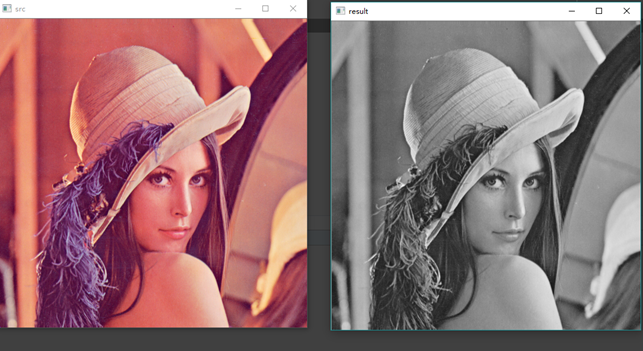（2）BRG通道转RGB通道

代码如下：

# encoding:utf-8
import cv2

# 读取图片

# 图像类型转换
result = cv2.cvtColor(src, cv2.COLOR_BGR2RGB)

# 显示图像
cv2.imshow("src", src)
cv2.imshow("result", result)

# 等待显示
cv2.waitKey(0)
cv2.destroyAllWindows()

运行结果如下：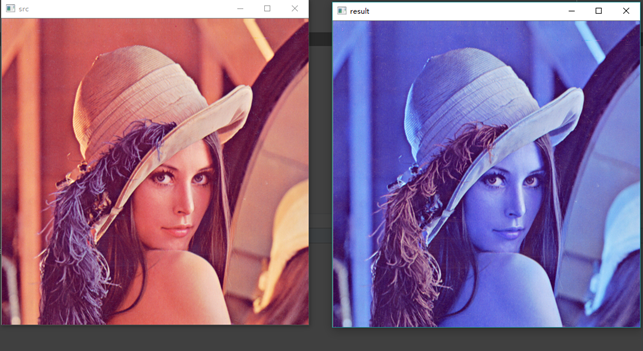一般在图像处理中，彩色图像转灰度图像最常用。

参考资料

 https://blog.csdn.net/Eastmount/article/details/82347501

 Python+OpenCV图像处理

展开全文数字图像处理 Python 加法运算 类型转换
• ## 图像为什么能相加

千次阅读 2017-12-23 22:34:39
我们知道2个矩阵是可以执行加法运算的，那2个图像能不能相加呢？当然是可以的。前面提到，图像就是函数，函数可以相加图像当然也可以相加。我们知道2个矩阵相加必须是同一维度的，即行列相同，图像当然也一样，即...
我们知道2个矩阵是可以执行加法运算的，那2个图像能不能相加呢？当然是可以的。前面提到，图像就是函数，函数可以相加，图像当然也可以相加。

我们知道2个矩阵相加必须是同一维度的，即行列相同，图像当然也一样，即宽度和高度相同。

我们看下面2副图像：import cv2
print(boats.shape)
print(goldhill.shape)

输出结果：

(576, 720)
(576, 720)

可以看出，这2副图像的宽度和高度分别是720和576，大小是一致的。2个图像相加就是2个矩阵相加。

unite = boats + goldhill
cv2.imshow("unite", unite)
cv2.waitKey()

输出结果：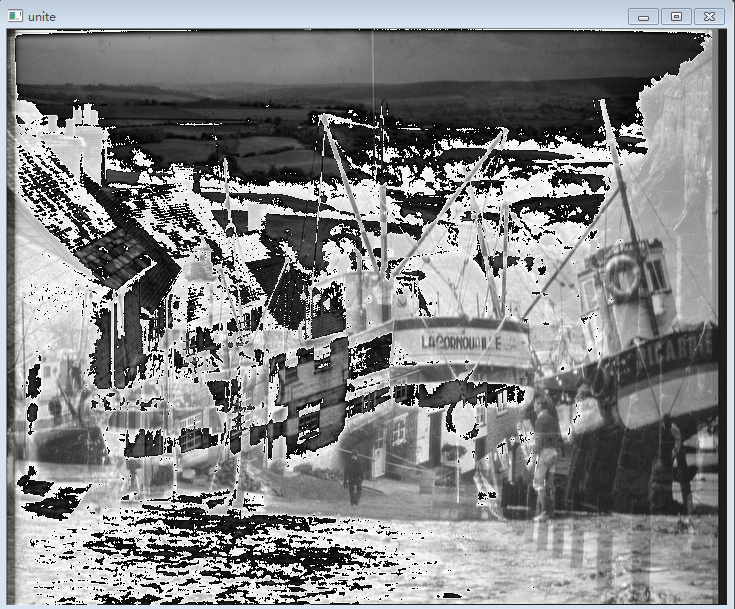不怎么样嘛！白块，黑块怎么那么多！有的地方变白了，有的地方变黑了。what’s wrong?

我们知道，图像的强度取值区间是[0, 255]，opencv的图像类型是uint8类型。而numpy的矩阵加法是一种模（mod）操作，即200+60=260 % 256 = 4，所以该亮的地方反而暗淡了。

另外，2副图像相加，我们实际上需要的2副图像相对位置强度的平均值，因此在相加前，需要各除以2，然后再相加（注意要整除）。

unite = cv2.add(boats // 2 , goldhill // 2)
cv2.imshow("unite", unite)
cv2.waitKey()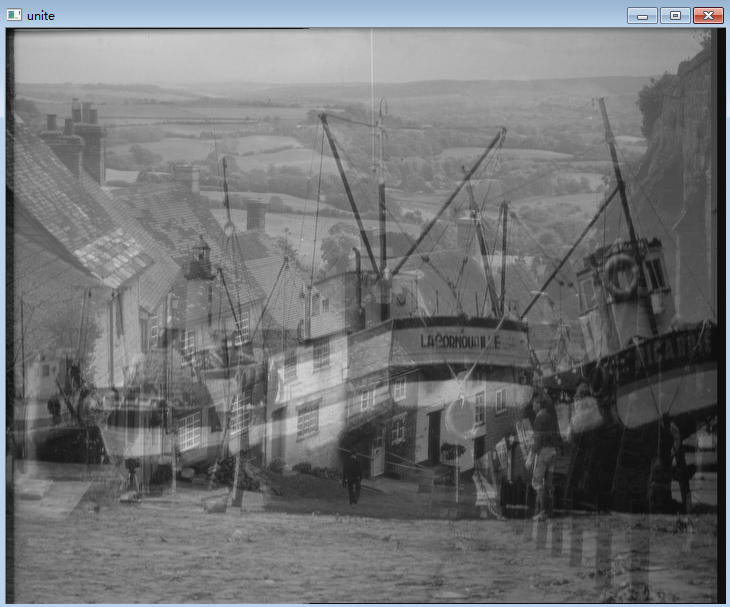我们知道，除以2和乘以0.5是等价的。乘以0.5是一个数乘运算，也就是说，图像自身还可以进行这种运算（注意乘以浮点数后矩阵类型发生了改变，而opencv的图像矩阵必须转换成为uint8类型才能正常显示）。

boats20 = 0.2 * boats
boats20 = boats20.astype("uint8")
cv2.imshow("boats20", boats20)
cv2.waitKey()

输出结果：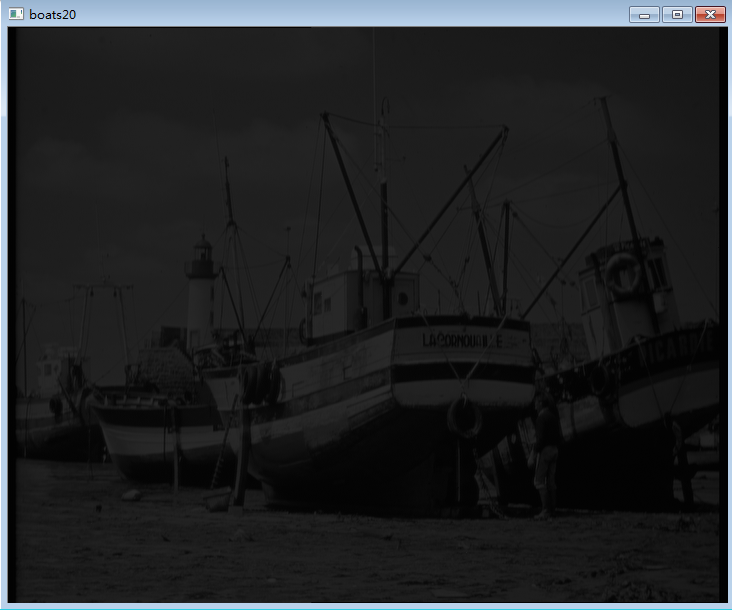可以看到，整体效果暗淡了很多。因为所有的像素点的值都乘以了0.2，强度变成了原来的20%。如果乘的数值小于1，图像整体就变暗，如果乘的数值大于1，整体就变亮。

这给了我们混合2张图像的一种比率调和方法，例如最前面2张图，我们想要看到更多的船（注意为确保2张图像的混合值小于255，系数之和应保持等于1）。

unite = 0.75 * boats + 0.25 * goldhill
unite = unite.astype("uint8")
cv2.imshow("unite", unite)
cv2.waitKey()()。代码如下，效果是一样的。

unite = cv2.addWeighted(boats, 0.75, goldhill, 0.25, 0)
cv2.imshow("unite", unite)
cv2.waitKey()

那么：图像为什么要相加呢？为了混合(blend)2张图像。

实质上，图像相加还有一个用途：降噪。同一场景的不同时间采集图像通过相加平均后去除噪声，这个后面再讲。
展开全文图像处理 opencv 图像相加
• 由于这段时间在做一个图像处理与三维重建的项目，其中数字图像处理的知识是必不可少的，学习matlab也有一段时间了，所以现在抽点时间将这段时间所学的东西做个小总结，加深理解的同时也方便以后有需要时可以查看一下...图像处理 matlab
• 数字图像处理(Digital Image Processing)是通过计算机对图像进行去除噪声、增强、复原、分割、提取特征等处理的方法和技术。本专栏将以学习笔记形式对数字图像处理的重点基础知识进行总结整理，欢迎大家一起学习交流...数字图像处理
• 数字图像处理是指将图像信号转换成数字信号并利用计算机对其进行处理的过程。图像处理最早出现于 20 世纪 50 年代，当时的电子计算机已经发展到一定水平，人们开始利用计算机来处理图形和图像信息。数字图像处理作为...
• 所谓数字图像处理，是指将图像信号转换成数字信号并利用计算机对其进行处理的过程。20 世纪 50 年代，电子计算机已经发展到一定水平，人们开始利用计算机来处理图形和图像信息，这便是早期的图像处理。早期图像处理...
• 第 1 章　基本的图像操作和处理本章讲解操作和处理图像的基础知识，将通过大量示例介绍处理图像所需的 Python 工具包，并介绍用于读取图像、图像转换和缩放、计算导数、画图和保存结果等的基本工具。这些工具的使用...
• 数字图像处理第六章数字图像处理---彩色图像处理（五） 彩色图像的空间滤波5.1 彩色图像的平滑处理5.2 彩色图像的锐化处理（六） 直接在 RGB 矢量空间中 处理6.1 使用梯度的彩色边缘检测6.2 在 RGB向量空间中分割...彩色图像处理 平滑处理
• ## 图像处理与识别学习小结

万次阅读 热门讨论 2009-08-31 23:14:00
图像处理与识别学习小结 数字图像处理是对图像进行分析、加工、和处理，使其满足视觉、心理以及其他要求的技术。图像处理是信号处理在图像域上的一个应用。目前大多数的图像是以数字形式存储，因而图像处理很多情况...图像处理 processing 算法 image
• ## 图像处理与识别

千次阅读 2016-07-01 08:45:03
图像处理与识别学习小结   数字图像处理是对图像进行分析、加工、和处理，使其满足视觉、心理以及其他要求的技术。图像处理是信号处理在图像域上的一个应用。目前大多数的图像是以数字形式存储，因而图像处理很多...
• 本文主要讲述基于VC++6.0 MFC图像处理的应用知识，主要结合自己大三所学课程《数字图像处理》及课件进行讲解，主要通过MFC单文档视图实现显示BMP图像增强处理，包括图像普通平滑、高斯平滑、不同算子的图像锐化知识...图像处理 图像增强
• 数字图像处理第六章数字图像处理---彩色图像处理（一） 在 MATLAB 中彩色图像的表示（二）仿射变换（三）投影变换（四）应用于图像的几何变换（五）MATLAB 中的图像坐标系统5.1 输出图像位置5.2 控制输出网格（六）...彩色图像处理 HSV 彩色空间
• 本文主要讲解图像傅里叶变换的相关内容，在数字图像处理中，有两个经典的变换被广泛应用——傅里叶变换和霍夫变换。其中，傅里叶变换主要是将时间域上的信号转变为频率域上的信号，用来进行图像除噪、图像增强等处理...Python图像处理 傅里叶变换 OpenCV 傅里叶逆变换
• 代数运算-图像相加，就是对两幅图像的点之间进行加的运算。四种运算相应的公式为：  s(x,y) = f(x,y) + g(x,y)  图像相加常用来求平均值去除addtive噪声或者实现二次曝光（double-exposure）。
• ## 图像处理岗位面试题搜罗汇总

万次阅读 多人点赞 2018-06-21 15:11:46
原文...传统图像处理部分 图像处理基础知识 彩色图像、灰度图像、二...
...